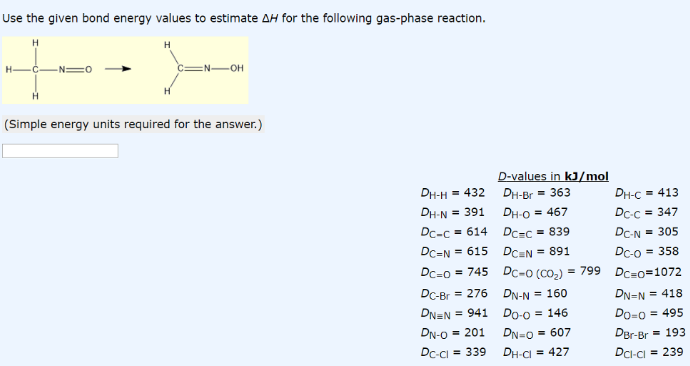# Problem: Use the given bond energy values to estimate ΔH for the following gas-phase reaction. (Simple energy units required for the answer.)

###### FREE Expert Solution
85% (255 ratings)###### Problem Details

Use the given bond energy values to estimate ΔH for the following gas-phase reaction. (Simple energy units required for the answer.)